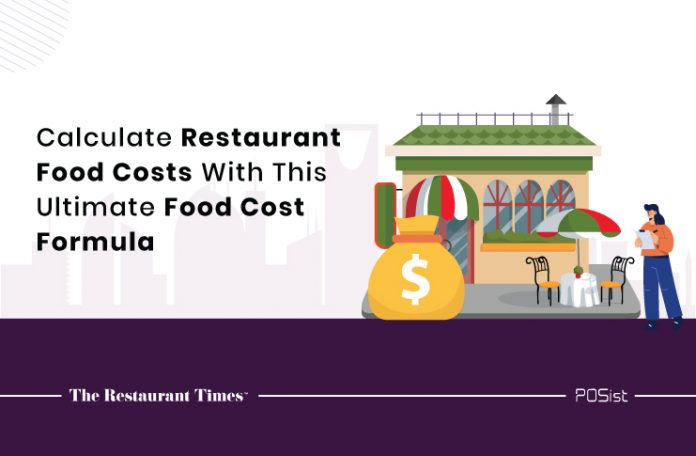The Ultimate Food Cost Formula For Saudi Arabia RestaurateursFood cost control is one of the significant priorities of running a restaurant. Irrespective of the geographic location, uncontrolled food costs can create problems for all types of restaurants. Regular and accurate calculation of food cost is thus necessary for restaurant operators to keep the business afloat. Owing to the thin profit margins of restaurants, using a food cost formula that gives accurate cost proportions of everything from raw material to preparation is imperative.

Many reasons make it essential to calculate food costs. Some of these are:

Often, restaurant operators overlook the importance of food cost control as they might find collating all the costs together and calculating the profit margins problematic. However, if not done right, food costs might result in more losses than profits. Having a well-defined food cost formula makes things easier for the operators.

This article will present the best practices for calculating food cost to keep your food costs in check.

What Is The Food Cost Formula?

The food cost formula is a formula that shows the variation between your actual food costs and the ideal food costs. The variation could be due to wastage, thefts, and other factors. The ideal food costs are determined by considering an ideal restaurant with no wastage, while the actual food costs are determined taking into account the real picture of the restaurant.

The ideal food costs give the restaurant operators a benchmark to compare their own food costs and make adjustments. If the actual food costs are more than the benchmark, then it’s time to make a few amendments in the operating methods to reduce the variation.

How To Calculate Food Costs With A Food Cost Formula?

It is pretty easy to calculate the food costs with the help of the food cost formula. One needs to follow the following steps:

1. Calculate the actual food costs.
2. Calculate the ideal food costs.
3. Compare the actual and ideal food costs.

Let us look at how we can calculate the food costs step by step.

Calculating The Actual Food Cost

The actual food cost can be calculated by determining the inventory usage and the food sales. The formula for the same is:

Actual food costs = Inventory Usage/Total Food Sales, where

Inventory usage is the price of the inventory used in the week for a particular dish, and

Total food sales is the revenue of the total number of sales of that dish in the entire week.

Inventory usage is calculated considering the inventory on the first day of the week, the new inventory bought in the course of the week, and the inventory left on the last day of the week. Therefore, Inventory usage = Beginning inventory + New inventory purchases – Ending inventory.

For example,

Let us calculate the cost of roast chicken over a week. The beginning inventory of your kitchen was worth 400 SAR, you bought new inventory for 100 SAR, and you were left with 300 SAR worth of inventory on the last day of the week.

This makes your Inventory Usage = 400 SAR + 100 SAR – 300 SA = 200 SAR

Total food sales of the dish determine the overall revenue that it made. It is calculated based on the number of units sold. Thus, Total food sales = Sales Price of the dish * Number of units (plates) sold.

If the sales price or MRP of the dish is 25 SAR, and you sold 20 units of the dish that week, then the total food sales price becomes:

Total food sales = 25 SAR * 20 Units = 500 SAR

The actual food cost percentage for this example will be:

Actual Food Cost Percentage = Inventory Usage/Total Food Sales * 100 = 200/500 * 100 = 40 percent

The actual food cost percentage here, i.e., 40 percent, signifies that the cost to prepare the dish is on average 40 percent of its sales price. This cost includes all the wastage and spills that occur while preparing the dish. Next, we will calculate the ideal food costs.

Calculating The Ideal Food Costs

Ideal food costs are the costs incurred with no spillage or wastage of inventory. These costs explain the difference between the ideal and actual food costs, thereby helping the restaurateurs bridge the gap between both. Ideal food costs are calculated on the basis of the cost of ingredients and the sales price of the dish. The formula to calculate ideal food costs is: Ideal Food Costs = Recipe Cost (Ingredient wise)/Recipe Sales Cost (MRP)

Recipe cost is the accumulated cost of every ingredient used to prepare the dish. To continue with our example, let us assume the roast chicken is made of chicken and buffalo sauce. 1kg of chicken and 1 bottle of buffalo sauce make 4 units of the dish. The cost of 1kg of chicken is 18 SAR, while that of 1 bottle of buffalo sauce is 10 SAR. Thus, the cost of 4 units ingredient-wise would be 18 SAR + 10 SAR = 28 SAR. This would make the cost of a single unit of roast chicken 28 SAR / 4 units = 7 SAR. This is the recipe cost of the roast chicken.

Recipe Sales Cost, i.e., the MRP of the dish is 25 SAR, as mentioned above.

Thus, Ideal Food Cost Percentage = 7/25 * 100 = 28 percent